Anúncio

# Fibonaaci sequence.pptx

8 de Feb de 2023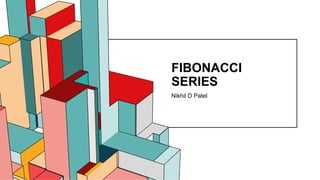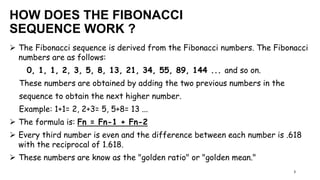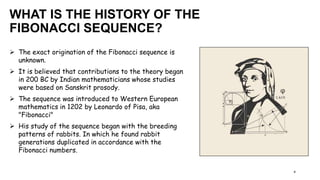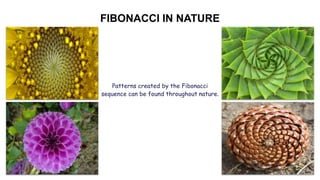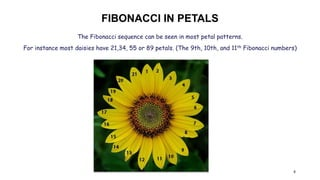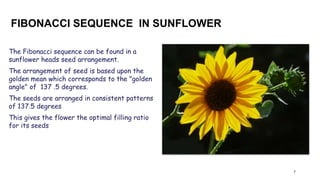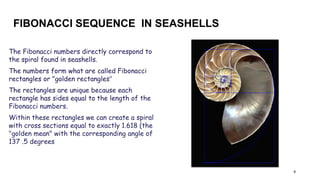1 de 9
Anúncio

### Fibonaaci sequence.pptx

1. 6.53 FIBONACCI SERIES Nikhil D Patel
2. WHAT IS FIBONACCI SERIES? 2  The Fibonacci sequence is a series of numbers that follow a unique integer sequence.  These numbers generate mathematical patterns that can be found in all aspects of life.  The patterns can be seen in everything from the human body to the physiology of plants and animals.
3. HOW DOES THE FIBONACCI SEQUENCE WORK ? 3  The Fibonacci sequence is derived from the Fibonacci numbers. The Fibonacci numbers are as follows: 0, 1, 1, 2, 3, 5, 8, 13, 21, 34, 55, 89, 144 ... and so on. These numbers are obtained by adding the two previous numbers in the sequence to obtain the next higher number. Example: 1+1= 2, 2+3= 5, 5+8= 13 ...  The formula is: Fn = Fn-1 + Fn-2  Every third number is even and the difference between each number is .618 with the reciprocal of 1.618.  These numbers are know as the "golden ratio" or "golden mean."
4. WHAT IS THE HISTORY OF THE FIBONACCI SEQUENCE? 4  The exact origination of the Fibonacci sequence is unknown.  It is believed that contributions to the theory began in 200 BC by Indian mathematicians whose studies were based on Sanskrit prosody.  The sequence was introduced to Western European mathematics in 1202 by Leonardo of Pisa, aka "Fibonacci"  His study of the sequence began with the breeding patterns of rabbits. In which he found rabbit generations duplicated in accordance with the Fibonacci numbers.
5. FIBONACCI IN NATURE 5 Patterns created by the Fibonacci sequence can be found throughout nature.
6. FIBONACCI IN PETALS 6 The Fibonacci sequence can be seen in most petal patterns. For instance most daisies have 21,34, 55 or 89 petals. (The 9th, 10th, and 11th Fibonacci numbers)
7. FIBONACCI SEQUENCE IN SUNFLOWER 7 The Fibonacci sequence can be found in a sunflower heads seed arrangement. The arrangement of seed is based upon the golden mean which corresponds to the "golden angle" of 137 .5 degrees. The seeds are arranged in consistent patterns of 137.5 degrees This gives the flower the optimal filling ratio for its seeds
8. FIBONACCI SEQUENCE IN SEASHELLS 8 The Fibonacci numbers directly correspond to the spiral found in seashells. The numbers form what are called Fibonacci rectangles or "golden rectangles" The rectangles are unique because each rectangle has sides equal to the length of the Fibonacci numbers. Within these rectangles we can create a spiral with cross sections equal to exactly 1.618 (the "golden mean" with the corresponding angle of 137 .5 degrees
9. THANK YOU
Anúncio